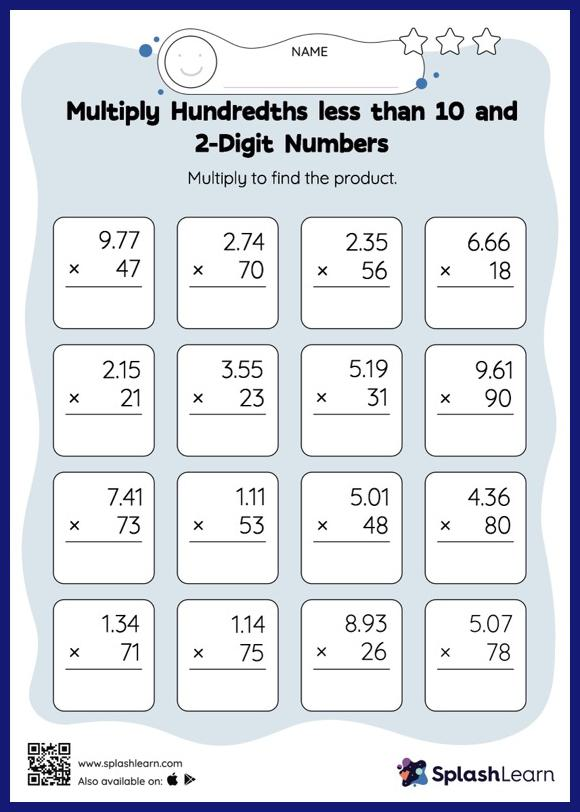# Multiply Hundredths less than 10 and 2-Digit Numbers: Vertical Multiplication Worksheet

Home > Multiply Hundredths less than 10 and 2-Digit Numbers: Vertical MultiplicationWhen multiplying a decimal by a two-digit number, students may ignore the decimal point during calculations and bring it back when writing the result. This multiply hundredths less than 10 and 2-digit numbers worksheet provides plenty of practice with this concept. In this worksheet, students practice solving problems using the column method. This method is especially helpful with problems involving bigger multi-digit numbers as the format provides an easy structure to follow.